Diversification is always good. It’s just limited in how much good it can do.

Diversification is achieved by adding assets into a portfolio which have correlations less than 1 with the portfolio. At its purest level, it reduce risk because not all assets will have the same gain or loss at the same time. By investing in different assets (which all have the same risk and return), we reduce the extreme movements of the portfolio, often in a way which doesn’t reduce the overall return quite as much.

To highlight how this works, let’s take an investment with an expected return of 6, and volatility of 12 (all example assets have these values in this post). We’ll mix the this asset equally with a clone of it, which is completely uncorrelated with the first asset. This reduces the volatility to 8.6 from 11 – a reduction in risk of 22%. The graph below illustrates what happens when we continue to add in more uncorrelated assets exactly like the first. The return stays constant, but the volatility continues to go down. But each time it goes down, it goes down a bit less.

[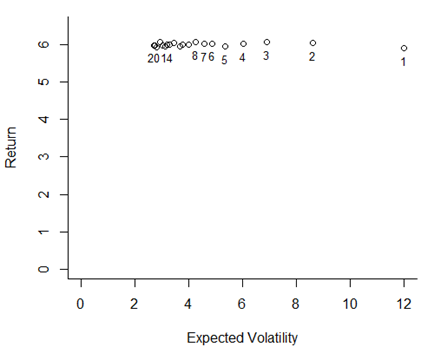We can look at this directly, and note how the decrease in volatility per increase in assets behaves. The ability to reduce risk falls off quickly, and we appear to hit a limit at a volatility of about 2.7.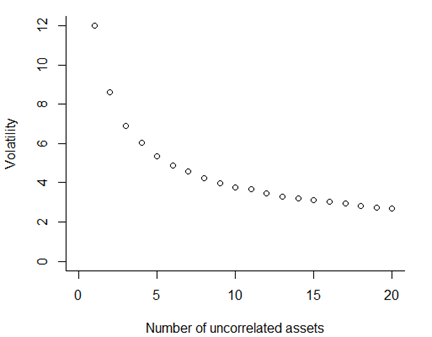The examples above give a very simple example of how diversification works, but they are unrealistic in a few ways. First, the assets are completely uncorrelated. It is almost impossible to find assets which are completely uncorrelated in the real world. Let’s run the same analysis again, but this time with a correlation of 0.5 across the assets.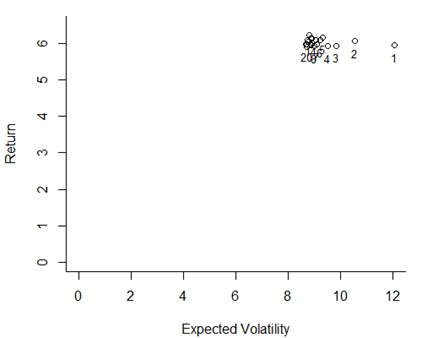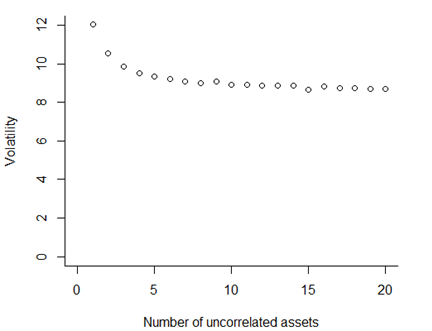Now we see that the benefits of diversification are strongly related to the (lack of) correlation between assets. If we run this analysis across all levels of correlation, and approximate the minimum level of volatility we can achieve, we get a graph like the one below. From a set of assets which all have an individual volatility of 12, we can reduce portfolio level volatility down to 2.7, but only if we have 20 uncorrelated assets. If the correlations rises to 0.5, our minimum volatility is much higher, at about 8.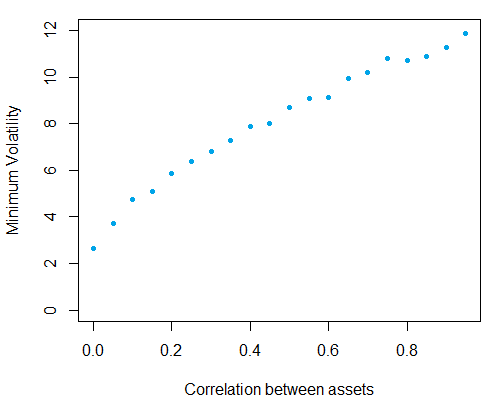So pure diversification – including uncorrelated assets with the same volatility level- always does help reduce volatility, but it the degree to which it helps depends on the correlations. And even completely uncorrelated assets have their limits. Given that the correlations amongst equity markets worldwide tend to be quite high - about `0.7 - 0.8` - we need to set our expectations about what diversification can achieve realistically.